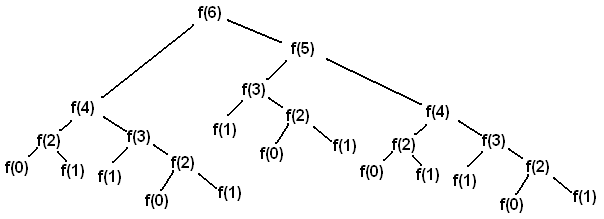## PROFILING - Profiling

Profiling: In software engineering, profiling ("program profiling", "software profiling") is a form of dynamic program analysis that measures, for example, the space (memory) or time complexity of a program, the usage of particular instructions, or the frequency and duration of function calls. Most commonly, profiling information serves to aid program optimization.

Wikipedia

Arthur and Jacob are newly introduced to the programming world and they are trying hard for Newbies contest. Yesterday, they learned about recursive functions and Fibonacci sequence. They tried to implement the function themselves so they wrote the following code:

int fibonacci (int N)

{

if(N < 2)

return N;

return fibonacci(N - 1) + fibonacci(N - 2);

}

But this program works slowly when N is a large number. They traced the program and found the cause of this problem. Take a look at the following picture:If you want to calculate Fibonacci(6), Fibonacci(3) will be calculated multiple times!

They want to know how serious this problem can be, so they need a profiler to calculate such a thing for them.

Your task is to provide the profiler which receives two integers N, K and tells them if they call Fibonacci(N) how many times Fibonacci(K) will be calculated (according to their code).

### Input

The first line of input indicates the number of test cases (There will be at most 100 test cases)

For each test case, there are two space-separated integers N, K  in a single line. (0≤N,K≤105)

### Output

For each test case, print the number of times Fibonacci(K) will be calculated, if we call Fibonacci(N). Since the result can be very large, print the result modulo (Mod %) 1000000007.

### Example

```Input:
5
6 6
6 3
6 2
100000 3
5 10

Output:
1
3
5
855252772
0```mrm_196: 2019-03-12 08:35:54 @fading: of course you should. If this algorithm was fast enough in the first place, there was no need to use a profiler :Dfading: 2018-12-18 18:07:49 With author's fibonaci algorithm, when N = 10^5, my program exceeds the time limit? So may I use other algorithm to overcome this or what can I do ?DOT: 2018-08-17 23:12:06 Thanks @wangchong756, I'd missed that case.spaceman_spiff: 2018-06-13 18:08:13 Last edit: 2018-06-13 18:19:35mrm_196: 2018-05-23 08:17:29 the problem with the consraints is fixed, sorry for thatprakash1108: 2018-05-19 13:36:40 Thanks @wangchong756 DP nailed it xD:) Last edit: 2018-05-23 11:29:34praney_rai: 2018-05-19 12:27:19 You should specify constraints atleast -_-Vipul Srivastava: 2018-05-18 12:00:38 Last edit: 2018-05-18 16:29:21